## ML Aggarwal Class 10 Solutions for ICSE Maths Chapter 11 Section Formula Ex 11

These Solutions are part of ML Aggarwal Class 10 Solutions for ICSE Maths. Here we have given ML Aggarwal Class 10 Solutions for ICSE Maths Chapter 11 Section Formula Ex 11

More Exercises

Midpoint Calculator is used to find the midpoint between 2 line segments using the midpoint formula. Use our calculator to find accurate midpoints step by step.

Question 1.
Find the co-ordinates of the mid-point of the line segments joining the following pairs of points:
(i) (2, – 3), ( – 6, 7)
(ii) (5, – 11), (4, 3)
(iii) (a + 3, 5b), (2a – 1, 3b + 4)
Solution:
(i) Co-ordinates of the mid-point of (2, -3), ( -6, 7)
$$\left( \frac { { x }_{ 1 }+{ x }_{ 2 } }{ 2 } ,\frac { { y }_{ 1 }+{ y }_{ 2 } }{ 2 } \right) or$$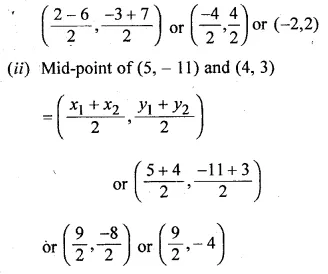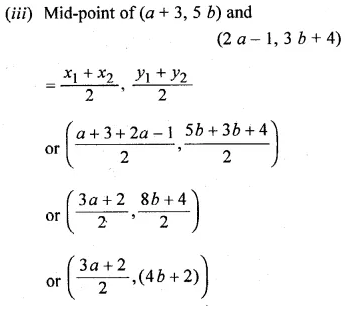Question 2.
The co-ordinates of two points A and B are ( – 3, 3) and (12, – 7) respectively. P is a point on the line segment AB such that AP : PB = 2 : 3. Find the co-ordinates of P.
Solution:
Points are A (-3, 3), B (12, -7)
Let P (x1,  y1) be the point which divides AB in the ratio of m1 : m2 i.e. 2 : 3
then co-ordinates of P will be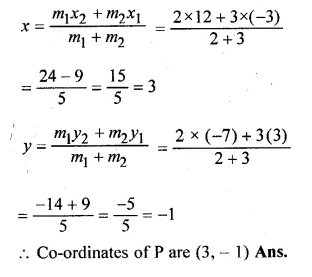Question 3.
P divides the distance between A ( – 2, 1) and B (1, 4) in the ratio of 2 : 1. Calculate the co-ordinates of the point P.
Solution:
Points are A (-2, 1) and B (1, 4) and
Let P (x, y) divides AB in the ratio of m1 : m2 i.e. 2 : 1
Co-ordinates of P will be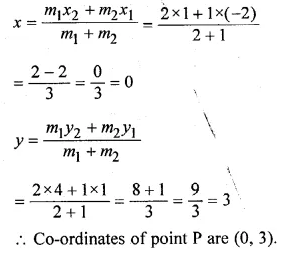Question 4.
(i) Find the co-ordinates of the points of trisection of the line segment joining the point (3, – 3) and (6, 9).
(ii) The line segment joining the points (3, – 4) and (1, 2) is trisected at the points P and Q. If the coordinates of P and Q are (p, – 2) and $$\left( \frac { 5 }{ 3 } ,q \right)$$ respectively, find the values of p and q.
Solution:
(i) Let P (x1, y1) and Q (x2, y2) be the points
which trisect the line segment joining the points
A (3, -3) and B (6, 9)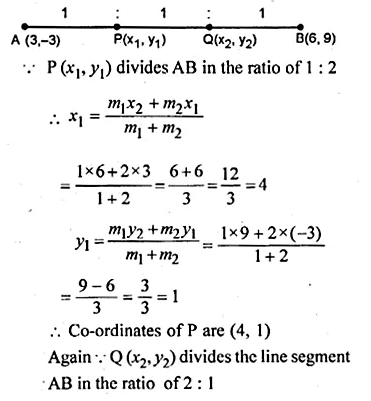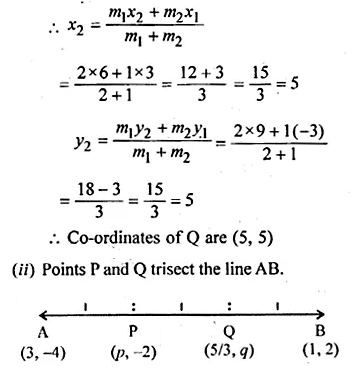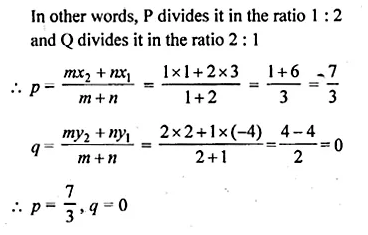Question 5.
(i) The line segment joining the points A (3, 2) and B (5, 1) is divided at the point P in the ratio 1 : 2 and it lies on the line 3x – 18y + k = 0. Find the value of k.
(ii) A point P divides the line segment joining the points A (3, – 5) and B ( – 4, 8) such that $$\frac { AP }{ PB } =\frac { k }{ 1 }$$ If P lies on the line x + y = 0, then find the value of k.
Solution:
(i) The point P (x, y) divides the line segment joining the points
A (3, 2) and B (5, 1) in the ratio 1 : 2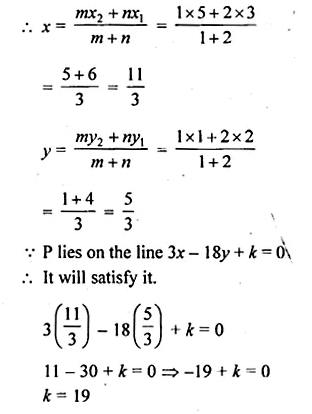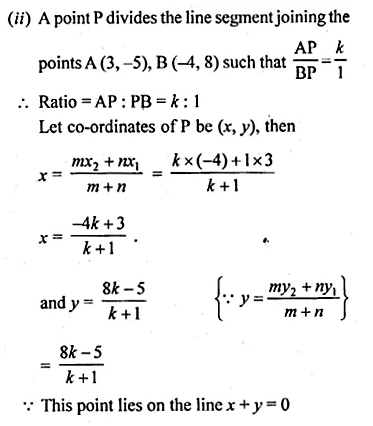Question 6.
Find the coordinates of the point which is three-fourth of the way from A (3, 1) to B ( – 2, 5).
Solution:
Let P be the required point, then
$$\frac { AP }{ AB } =\frac { 3 }{ 4 }$$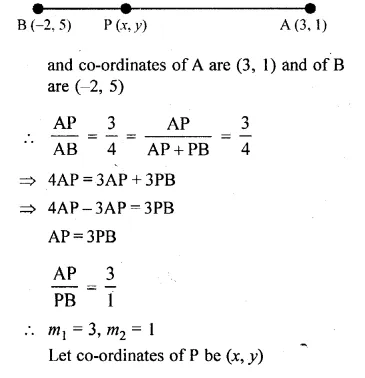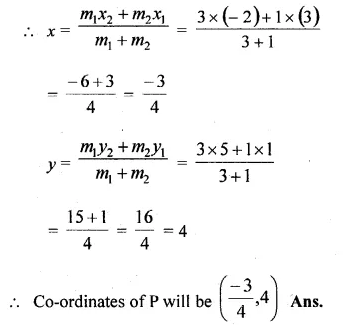Question 7.
Point P (3, – 5) is reflected to P’ in the x- axis. Also P on reflection in the y-axis is mapped as P”.
(i) Find the co-ordinates of P’ and P”.
(ii) Compute the distance P’ P”.
(iii) Find the middle point of the line segment P’ P”.
(iv) On which co-ordinate axis does the middle point of the line segment P P” lie ?
Solution:
(i) Co-ordinates of P’, the image of P (3, -5)
when reflected in x-axis will be (3, 5)
and co-ordinates of P”, the image of P (3, -5)
when reflected in y-axis will be (-3, -5)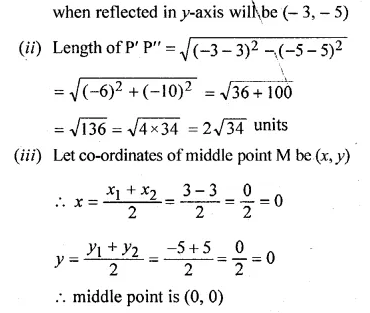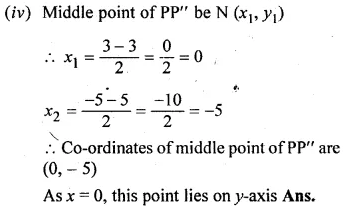Question 8.
Use graph paper for this question. Take 1 cm = 1 unit on both axes. Plot the points A(3, 0) and B(0, 4).
(i) Write down the co-ordinates of A1, the reflection of A in the y-axis.
(ii) Write down the co-ordinates of B1, the reflection of B in the x-axis.
(iii) Assign.the special name to the quadrilateral ABA1B1.
(iv) If C is the mid point is AB. Write down the co-ordinates of the point C1, the reflection of C in the origin.
(v) Assign the special name to quadrilateral ABC1B1.
Solution:
Two points A (3, 0) and B (0,4) have been plotted on the graph.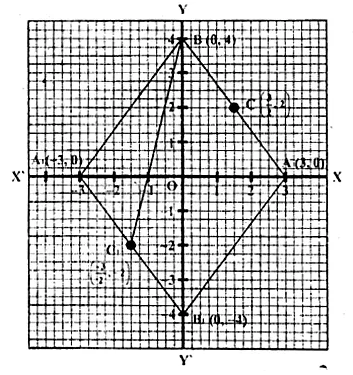(i)∵ A1 is the reflection of A (3, 0) in the v-axis Its co-ordinates will be ( -3, 0)
(ii)∵ B1 is the reflection of B (0, 4) in the .x-axis co-ordinates of B, will be (0, -4)
(iii) The so formed figure ABA1B1 is a rhombus.
(iv) C is the mid point of AB co-ordinates of C” will be $$\frac { AP }{ AB } =\frac { 3 }{ 4 }$$
∵ C, is the reflection of C in the origin
co-ordinates of C, will be $$\left( \frac { -3 }{ 2 } ,-2 \right)$$
(v) The name of quadrilateral ABC1B1 is a trapezium because AB is parallel to B1C1.

Question 9.
The line segment joining A ( – 3, 1) and B (5, – 4) is a diameter of a circle whose centre is C. find the co-ordinates of the point C. (1990)
Solution:
∵ C is the centre of the circle and AB is the diameter
C is the midpoint of AB.
Let co-ordinates of C (x, y)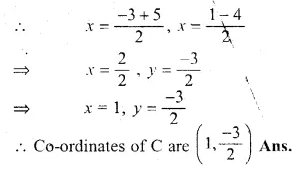Question 10.
The mid-point of the line segment joining the points (3m, 6) and ( – 4, 3n) is (1, 2m – 1). Find the values of m and n.
Solution:
Let the mid-point of the line segment joining two points
A(3m, 6) and (-4, 3n) is P( 1, 2m – 1)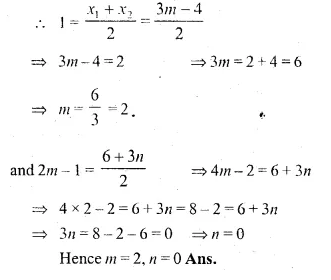Question 11.
The co-ordinates of the mid-point of the line segment PQ are (1, – 2). The co-ordinates of P are ( – 3, 2). Find the co-ordinates of Q.(1992)
Solution:
Let the co-ordinates of Q be (x, y)
co-ordinates of P are (-3, 2) and mid-point of PQ are (1, -2) then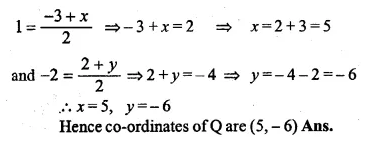Question 12.
AB is a diameter of a circle with centre C ( – 2, 5). If point A is (3, – 7). Find:
(i) the length of radius AC.
(ii) the coordinates of B.
Solution:
AC = $$\sqrt { { \left( 3+2 \right) }^{ 2 }+{ \left( -7-5 \right) }^{ 2 } }$$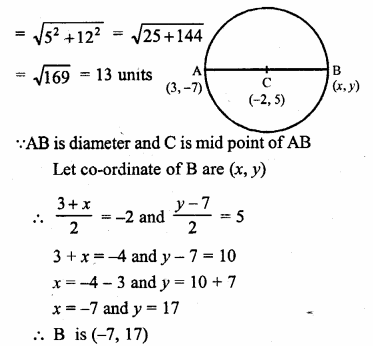Question 13.
Find the reflection (image) of the point (5, – 3) in the point ( – 1, 3).
Solution:
Let the co-ordinates of the images of the point A (5, -3) be
A1 (x, y) in the point (-1, 3) then
the point (-1, 3) will be the midpoint of AA1.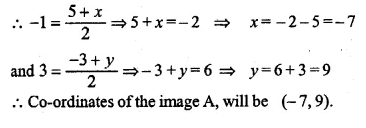Question 14.
The line segment joining A $$\left( -1,\frac { 5 }{ 3 } \right)$$ the points B (a, 5) is divided in the ratio 1 : 3 at P, the point where the line segment AB intersects y-axis. Calculate
(i) the value of a
(ii) the co-ordinates of P. (1994)
Solution:
Let P (x, y) divides the line segment joining
the points $$\left( -1,\frac { 5 }{ 3 } \right)$$, B(a, 5) in the ratio 1 : 3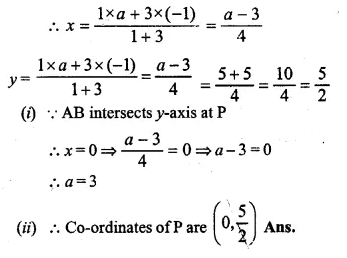Question 15.
The point P ( – 4, 1) divides the line segment joining the points A (2, – 2) and B in the ratio of 3 : 5. Find the point B.
Solution:
Let the co-ordinates of B be (x, y)
Co-ordinates of A (2, -2) and point P (-4, 1)
divides AB in the ratio of 3 : 5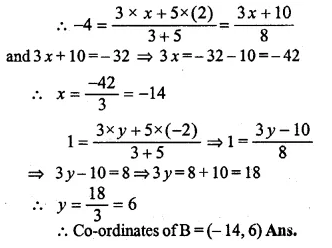Question 16.
(i) In what ratio does the point (5, 4) divide the line segment joining the points (2, 1) and (7 ,6) ?
(ii) In what ratio does the point ( – 4, b) divide the line segment joining the points P (2, – 2), Q ( – 14, 6) ? Hence find the value of b.
Solution:
(i) Let the ratio be m1 : m2 that the point (5, 4) divides
the line segment joining the points (2, 1), (7, 6).
$$5=\frac { { m }_{ 1 }\times 7+{ m }_{ 2 }\times 2 }{ { m }_{ 1 }+{ m }_{ 2 } }$$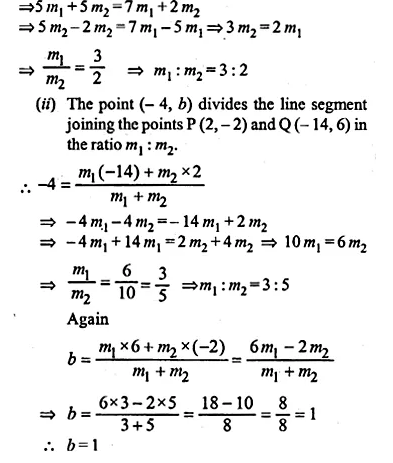Question 17.
The line segment joining A (2, 3) and B (6, – 5) is intercepted by the x-axis at the point K. Write the ordinate of the point k. Hence, find the ratio in which K divides AB. Also, find the coordinates of the point K.
Solution:
Let the co-ordinates of K be (x, 0) as it intersects x-axis.
Let point K divides the line segment joining the points
A (2, 3) and B (6, -5) in the ratio m1 : m2.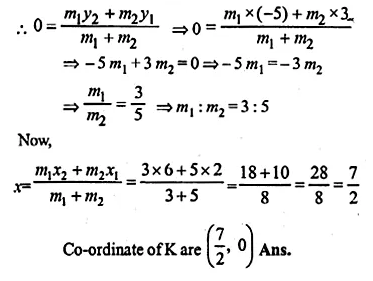Question 18.
If A ( – 4, 3) and B (8, – 6), (i) find the length of AB.
(ii) in what ratio is the line joining AB, divided by the x-axis? (2008)
Solution:
Given A (-4, 3), B (8, -6)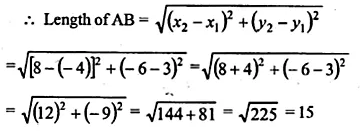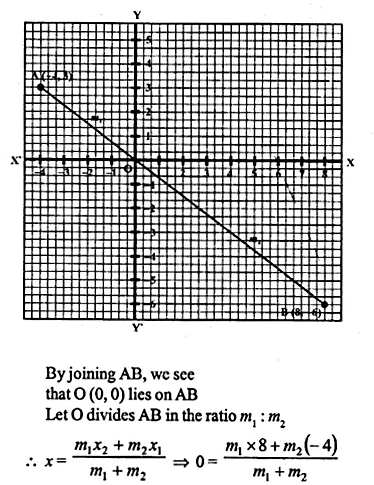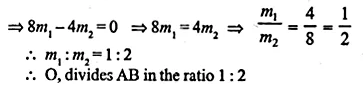Question 19.
(i) Calculate the ratio in which the line segment joining (3, 4) and( – 2, 1) is divided by the y-axis.
(ii) In what ratio does the line x – y – 2 = 0 divide the line segment joining the points (3, – 1) and (8, 9)? Also, find the coordinates of the point of division.
Solution:
(i) Let the point P divides the line segment joining the points
A (3, 4) and B (-2, 3) in the ratio of m1 : m2 and
let the co-ordinates of P be (0, y) as it intersects the y-axis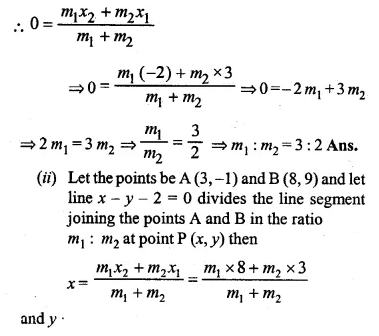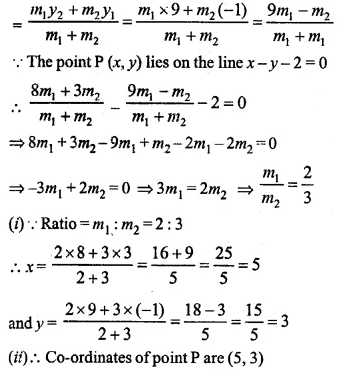Question 20.
Given a line segment AB joining the points A ( – 4, 6) and B (8, – 3). Find:
(i) the ratio in which AB is divided by the y-axis.
(ii) find the coordinates of the point of intersection.
(iii)the length of AB.
Solution:
(i) Let the y-axis divide AB in the ratio m : 1. So,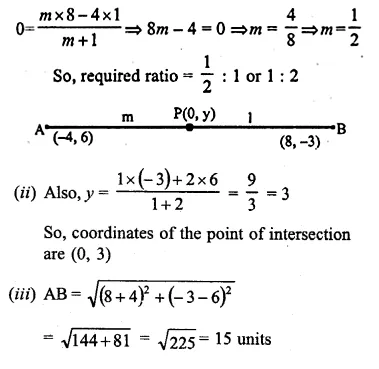Question 21.
(i) Write down the co-ordinates of the point P that divides the line joining A ( – 4, 1) and B (17,10) in the ratio 1 : 2.
(ii)Calculate the distance OP where O is the origin.
(iii)In what ratio does the y-axis divide the line AB ?
Solution:
(i) Let co-ordinate of P be (x, y) which divides the line segment joining the points
A ( -4, 1) and B(17, 10) in the ratio of 1 : 2.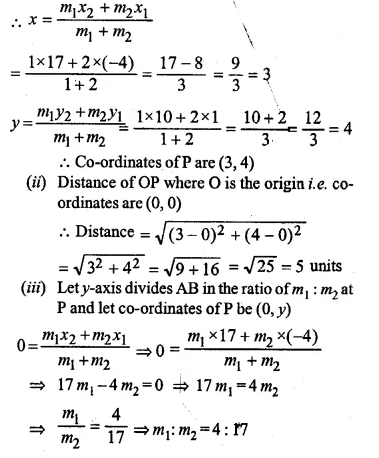Question 22.
Calculate the length of the median through the vertex A of the triangle ABC with vertices A (7, – 3), B (5, 3) and C (3, – 1)
Solution:
Let D (x, y) be the median of ΔABC through A to BC.
∴ D will be the midpoint of BC
∴ Co-ordinates of D will be,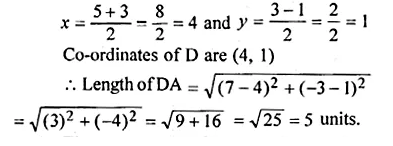Question 23.
Three consecutive vertices of a parallelogram ABCD are A (1, 2), B (1, 0) and C (4, 0). Find the fourth vertex D.
Solution:
Let O in the mid-point of AC the diagonal of ABCD
∴ Co-ordinates of O will be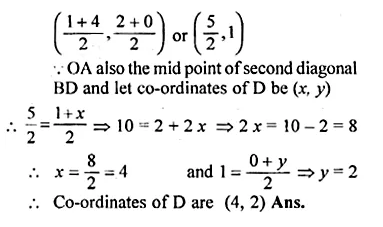Question 24.
If the points A ( – 2, – 1), B (1, 0), C (p, 3) and D (1, q) from a parallelogram ABCD, find the values of p and q.
Solution:
A (-2, -1), B (1, 0), C (p, 3) and D (1, q)
are the vertices of a parallelogram ABCD
∴ Diagonal AC and BD bisect each other at O
O is the midpoint of AC as well as BD
Let co-ordinates of O be (x, y)
When O is mid-point of AC, then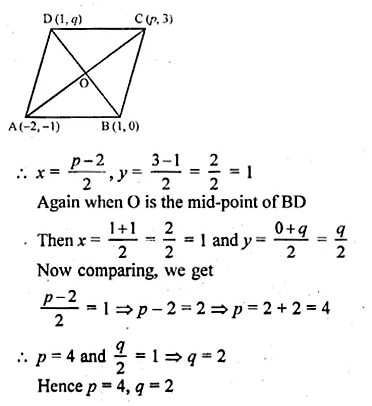Question 25.
If two vertices of a parallelogram are (3, 2) ( – 1, 0) and its diagonals meet at (2, – 5), find the other two vertices of the parallelogram.
Solution:
Two vertices of a ||gm ABCD are A (3, 2), B (-1, 0)
and point of intersection of its diagonals is P (2, -5)
P is mid-point of AC and BD.
Let co-ordinates of C be (x, y), then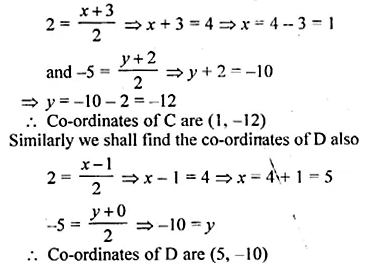Question 26.
Prove that the points A ( – 5, 4), B ( – 1, – 2) and C (5, 2) are the vertices of an isosceles right angled triangle. Find the co-ordinates of D so that ABCD is a square.
Solution:
Points A (-5, 4), B (-1, -2) and C (5, 2) are given.
If these are vertices of an isosceles triangle ABC then
AB = BC.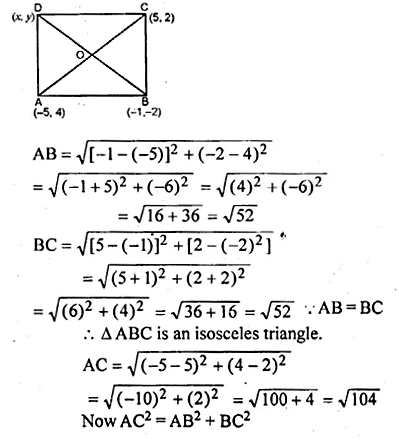Question 27.
Find the third vertex of a triangle if its two vertices are ( – 1, 4) and (5, 2) and mid point of one sides is (0, 3).
Solution:
Let A (-1, 4) and B (5, 2) be the two points and let D (0, 3)
be its the midpoint of AC and co-ordinates of C be (x, y).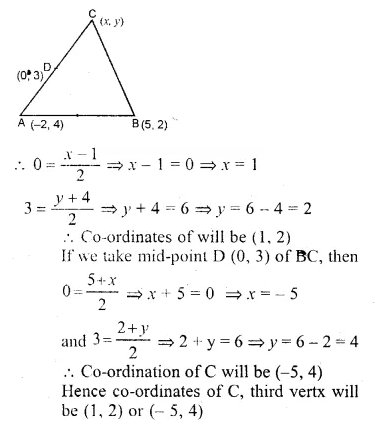Question 28.
Find the coordinates of the vertices of the triangle the middle points of whose sides are $$\left( 0,\frac { 1 }{ 2 } \right) ,\left( \frac { 1 }{ 2 } ,\frac { 1 }{ 2 } \right) and\left( \frac { 1 }{ 2 } ,0 \right)$$
Solution:
Let ABC be a ∆ in which $$D\left( 0,\frac { 1 }{ 2 } \right) ,E\left( \frac { 1 }{ 2 } ,\frac { 1 }{ 2 } \right) andF\left( \frac { 1 }{ 2 } ,0 \right)$$,
the mid-points of sides AB, BC and CA respectively.
Let co-ordinates of A be (x1, y1), B (x2, y2), C (x3, y3)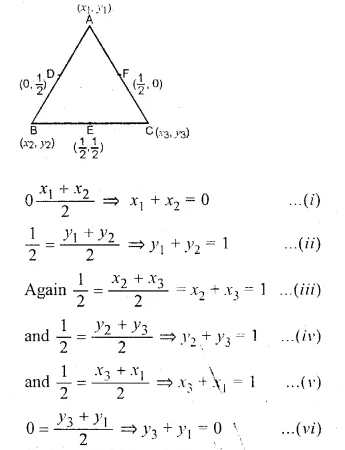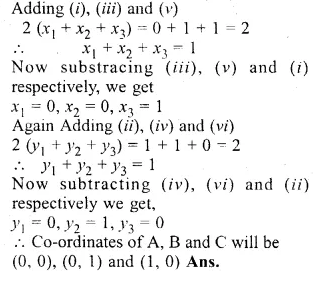Question 29.
Show by section formula that the points (3, – 2), (5, 2) and (8, 8) are collinear.
Solution:
Let the point (5, 2) divides the line joining the points (3, -2) and (8, 8)
in the ratio of m1 : m2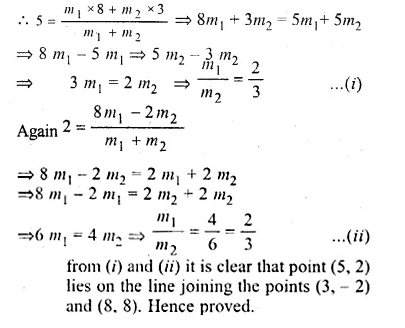Question 30.
Find the value of p for which the points ( – 5, 1), (1, p) and (4, – 2) are collinear.
Solution:
Let points A (-5, 1), B (1, p) and C (4, -2)
are collinear and let point A (-5, 1) divides
BC in the ratio in m1 : m2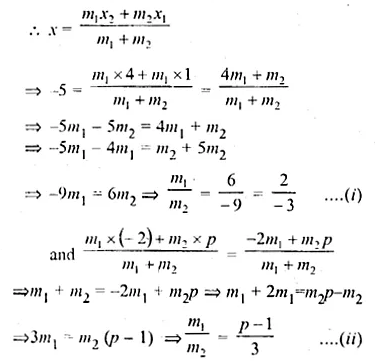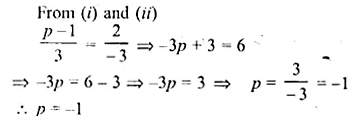Question 31.
A (10, 5), B (6, – 3) and C (2, 1) are the vertices of triangle ABC. L is the mid point of AB, M is the mid-point of AC. Write down the co-ordinates of L and M. Show that LM = $$\\ \frac { 1 }{ 2 }$$ BC.
Solution:
Co-ordinates of L will be
$$\left( \frac { 10+6 }{ 2 } ,\frac { 5-3 }{ 2 } \right) or\left( \frac { 16 }{ 2 } ,\frac { 2 }{ 2 } \right) or(8,1)$$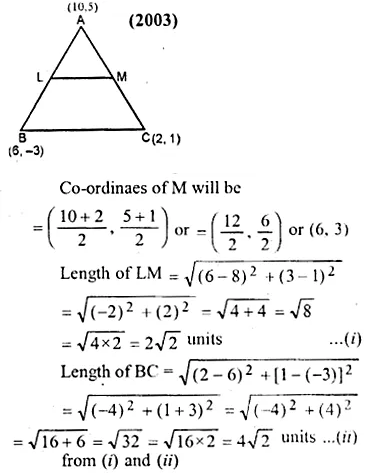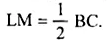Question 32.
A (2, 5), B ( – 1, 2) and C (5, 8) are the vertices of a triangle ABC. P and.Q are points on AB and AC respectively such that AP : PB = AQ : QC = 1 : 2.
(i) Find the co-ordinates of P and Q.
(ii) Show that PQ = $$\\ \frac { 1 }{ 3 }$$ BC.
Solution:
A (2, 5), B (-1, 2) and C (5, 8) are the vertices of a ∆ABC,
P and Q are points on AB
and AC respectively such that $$\frac { AP }{ PB } =\frac { AQ }{ QC } =\frac { 1 }{ 2 }$$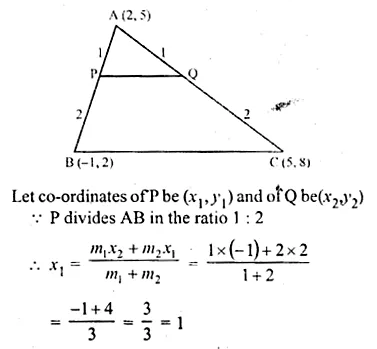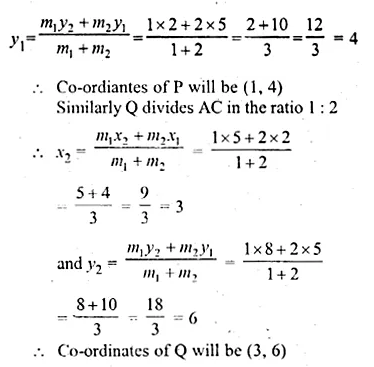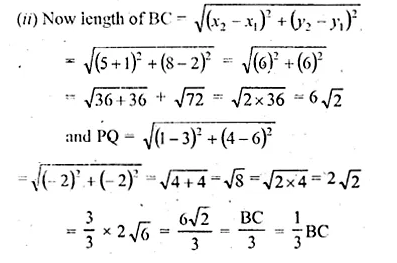Question 33.
The mid-point of the line segment AB shown in the adjoining diagram is (4, – 3). Write down die co-ordinates of A and B.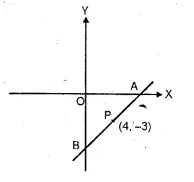Solution:
A lies on x-axis and B on the y-axis.
Let co-ordinates of A be (x, 0) and of B be (0, y)
P (4, -3) is the mid-point of AB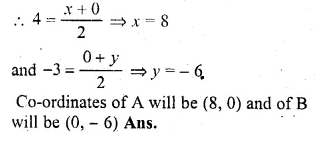Question 34.
Find the co-ordinates of the centroid of a triangle whose vertices are A ( – 1, 3), B(1, – 1) and C (5, 1) (2006)
Solution:
Co-ordinates of the centroid of a triangle,
whose vertices are (x1, y1), (x2, y2) and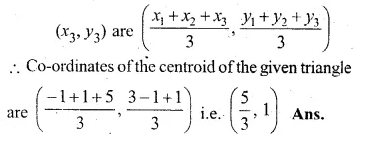Question 35.
Two vertices of a triangle are (3, – 5) and ( – 7, 4). Find the third vertex given that the centroid is (2, – 1).
Solution:
Let the co-ordinates of third vertices be (x, y)
and other two vertices are (3, -5) and (-7, 4)
and centroid = (2, -1).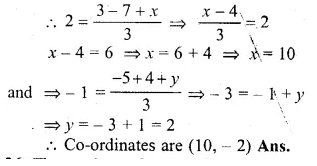Question 36.
The vertices of a triangle are A ( – 5, 3), B (p – 1) and C (6, q). Find the values of p and q if the centroid of the triangle ABC is the point (1, – 1).
Solution:
The vertices of ∆ABC are A (-5, 3), B (p, -1), C (6, q)
and the centroid of ∆ABC is O (1, -1)
co-ordinates of the centroid of ∆ABC will be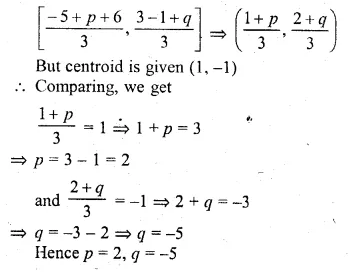Hope given ML Aggarwal Class 10 Solutions for ICSE Maths Chapter 11 Section Formula Ex 11 are helpful to complete your math homework.

If you have any doubts, please comment below. Learn Insta try to provide online math tutoring for you.

## Selina Concise Mathematics Class 10 ICSE Solutions 2020-21 Edition

Learninsta.com provides step by step solutions for Selina Concise ICSE Solutions for Class 10 Mathematics. You can download the Selina Concise Mathematics ICSE Solutions for Class 10 with Free PDF download option. Selina Publishers Books of Concise Mathematics for Class 10 ICSE Solutions all questions are solved and explained by expert mathematic teachers as per ICSE board guidelines. By studying these Selina ICSE Solutions for Class 10 Maths you can easily get good marks in ICSE Class 10 Board Examinations.

## Understanding ICSE Mathematics Class 10 ML Aggarwal Solved Solutions

Get Latest Edition of ML Aggarwal Class 10 Solutions PDF Download on LearnInsta.com. It provides step by step solutions for ML Aggarwal Maths for Class 10 ICSE Solutions Pdf Download. You can download the Understanding ICSE Mathematics Class 10 ML Aggarwal Solved Solutions with Free PDF download option, which contains chapter wise solutions. APC Maths Class 10 Solutions ICSE all questions are solved and explained by expert Mathematic teachers as per ICSE board guidelines. By studying these ML Aggarwal Class 10 ICSE Solutions you can easily get good marks in ICSE Class 10 Board Examinations. You also refer Selina Concise Mathematics Class 10 Solutions for more practice.

APC Understanding ICSE Mathematics Class 10 ML Aggarwal Solutions 2018 Edition for 2019 Examinations

ML Aggarwal Class 10 Maths Chapter 1 Value Added Tax

ML Aggarwal Class 10 Maths Chapter 2 Banking

ML Aggarwal Class 10 Maths Chapter 3 Shares and Dividends

ML Aggarwal Class 10 Maths Chapter 4 Linear Inequations

ML Aggarwal Class 10 Maths Chapter 5 Quadratic Equations in One Variable

ML Aggarwal Class 10 Maths Chapter 6 Factorization

ML Aggarwal Class 10 Maths Chapter 7 Ratio and Proportion

ML Aggarwal Class 10 Maths Chapter 8 Matrices

ML Aggarwal Class 10 Maths Chapter 9 Arithmetic and Geometric Progressions

ML Aggarwal Class 10 Maths Chapter 10 Reflection

ML Aggarwal Class 10 Maths Chapter 11 Section Formula

ML Aggarwal Class 10 Maths Chapter 12 Equation of a Straight Line

ML Aggarwal Class 10 Maths Chapter 13 Similarity

ML Aggarwal Class 10 Maths Chapter 14 Locus

ML Aggarwal Class 10 Maths Chapter 15 Circles

ML Aggarwal Class 10 Maths Chapter 16 Constructions

ML Aggarwal Class 10 Maths Chapter 17 Mensuration

ML Aggarwal Class 10 Maths Chapter 18 Trigonometric Identities

ML Aggarwal Class 10 Maths Chapter 19 Trigonometric Tables

ML Aggarwal Class 10 Maths Chapter 20 Heights and Distances

ML Aggarwal Class 10 Maths Chapter 21 Measures of Central Tendency

ML Aggarwal Class 10 Maths Chapter 22 Probability

### FAQs on ML Aggarwal Class 10 Solutions

1.  How do I download the PDF of ML Aggarwal Solutions in Class 10?

All you have to do is tap on the direct links available on our LearnInsta.com page to access the Class 10 ML Aggarwal Solutions in PDF format. You can download them easily from here for free of cost.

2. Where can I find the solutions for ML Aggarwal Maths Solutions for Class 10?

You can find the Solutions for ML Aggarwal Maths for Class 10 from our page. View or download them as per your convenience and aid your preparation to score well.

3. What are the best sources for Class 10 Board Exam Preparation?

Aspirants preparing for their Class 10 Board Exams can make use of the quick and direct links available on our website LearnInsta.com regarding ML Aggarwal Solutions.

4. Is solving ML Aggarwal Solutions Chapterwise benefit you during your board exams?

Yes, it can be of huge benefit during board exams as you will have indepth knowledge of all the topics by solving Chapterwsie ML Aggarwal Solutions.

Candidates can download the Class 10 Maths ML Aggarwal Solutions PDF from the direct links available on our page. We don’t charge any amount from you and they are absolutely free of cost.

## Selina Concise Mathematics Class 10 ICSE Solutions Chapter 3 Shares and Dividend Ex 3A

These Solutions are part of Selina Concise Mathematics Class 10 ICSE Solutions. Here we have given Selina Concise Mathematics Class 10 ICSE Solutions Chapter 3 Shares and Dividend Ex 3A.

Other Exercises

Question 1.
How much money will be required to buy 400, ₹ 12.50 shares at a premium of ₹ 1?
Solution:
Number of shares purchased = 400
Rate of each share = ₹ 12.50
M.V. = ₹ 1 premium = ₹ 12.50 + ₹ 1 = ₹ 13.50
Amount of in vestment = ₹ 400 x ₹ 13.50 = ₹ 5400

Question 2.
How much money will be required to buy 250, ₹ 15 shares at a discount of ₹ 1.50?
Solution:
Number of shares = 250
M.V. = at ₹ 15 at a discount of ₹ 1.50 = ₹ 15 – ₹ 1.50 = ₹ 13.50
Amount of investment = ₹ 13.50 x 250 = ₹ 3375

Question 3.
A person buys 120 shares at a nominal value of ₹ 40 each, which he sells at ₹ 42.50 each. Find his profit and profit percent.
Solution:
No. of shares = 120
Nominal value of each share = ₹ 40.00
Profit at each share = ₹ 42.50 – ₹ 40.00 = ₹ 2.50
Total profit = 2.50 x 120 = ₹ 300
Cost price of 120 shares = ₹ 40 x 120 = ₹ 4,800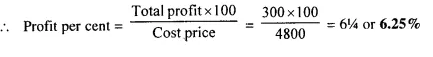Question 4.
Find the cost of 85 shares of Rs. 60 each when quoted at ₹ 63.25
Solution:
No. of shares = 85
Market value of cach share = ₹ 63.25
Total cost = ₹ 63.25 x 85 = ₹ 5,376.25

Question 5.
A man invests ₹ 800 in buying 75 shares and when they are selling at a premium of ₹ 1.15, he sells all the shares. Find his profit and profit percent.
Solution:
Investment = ₹ 800
In first case face value of each share = ₹ 5
and market value of each share = ₹ 5.00 + ₹ 1.15 = ₹ 6.15
Gain on each share of ₹ 5 = ₹ 1.15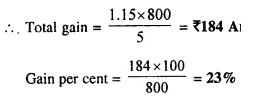Question 6.
Find the annual income derived from 125, ₹ 120 shares paying 5% dividend.
Solution:
Amount of investment = ?
Number of shares purchased = 125 at ₹ 120, 5% dividend
Amount of investment = ₹ 125 x 120 = ₹ 15000
His annual income = 15000 x $$\frac { 5 }{ 100 }$$ = ₹ 750

Question 7.
A man invests ₹ 3,072 in a company paying 5% per annum when its ₹ 10 share can be bought for ₹ 16 each. Find:
(i) his annual income;
(ii) his percentage income on his investment.
Solution:
Total investment = ₹ 3,072
Market value of each shares = ₹ 16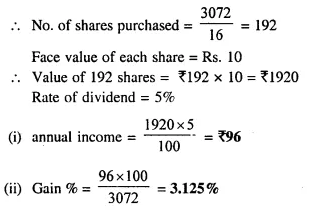Question 8.
A man invests ₹ 7,770 in a company paying 5 percent dividend when a share of nominal value of ₹ 100 sells at a premium of ₹ 5. Find :
(i) the number of shares bought;
(ii) annual income ;
(iii) percentage income ;
Solution:
Investment = ₹ 7770
Nominal value of each share = 100
Market value = 100 + 5 = 105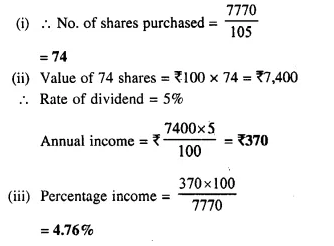Question 9.
A man buys ₹ 50 shares of a company paying 12 percent dividend, at a premium of ₹ 10. Find :
(i) the market value of 320 shares ;
(ii) his annual income ;
(iii) his profit percent.
Solution:
(i) Market value of each share = ₹ 50 + ₹ 10 = ₹ 60
Market value of 320 shares = ₹ 60 x 320 = ₹ 19,200
(ii) Rate of dividend = 12%
Face value of 320 shares = Rs. 50 x 320 = Rs. 16,000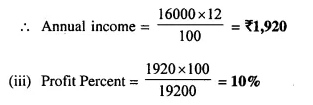Question 10.
A man buys of Rs. 75 shares at a discount of Rs. 15 of a company paying 20% dividend. Find :
(i) the market value of 120 shares ;
(ii) his annual income ;
(iii) his profit percent.
Solution:
(i) Market value of one share = Rs. 75 – 15 = Rs. 60
Market value of 120 shares = Rs. 60 x 120 = Rs. 7,200
(ii) Rate of dividend = 20%
Face value of 120 shares = Rs. 75 x 120 = Rs. 9,000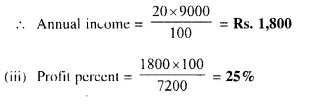Question 11.
A man has 300, ₹ 50 shares of a company paying 20% dividend. Find his net income after paying 3% income tax.
Solution:
No. of shares = 300
Face value of 50 shares = Rs. 50 x 300 = Rs. 15,000
Rate of dividend = 20%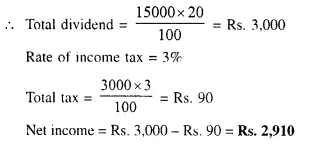Question 12.
A company pays dividend of 15 % on its ten-rupee shares from which it deducts income tax at the rate of 22%. Find the annual income of a man who owns one thousand shares of this company.
Solution:
No. of shares = 1,000
Face Value of each share = Rs. 10
Rate of dividend = 15%
Rate of income tax = 22%
Face value of 1,000 shares = 1,000 x 10 = Rs. 10,000
Total dividend = Rs. 10,000 x $$\frac { 15 }{ 100 }$$ = Rs. 1,500
Income tax deducted = Rs. 1500 x $$\frac { 22 }{ 100 }$$ = Rs. 330
Net income = Rs.1500 – Rs. 330 = Rs. 1170

Question 13.
A man invests Rs. 8,800 in buying shares of a company of face value of rupees hundred each at a premium of 10%. If he earns Rs. 1,200 at the end of the year as dividend find:
(i) the number of shares he has in the company;
(ii) the dividend percent per share. 
Solution:
Investment = Rs. 8,800
Face value of each share = Rs. 100
Market value of each share = Rs. 100 + 10 = Rs. 110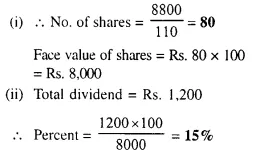Question 14.
A man invests Rs. 1,680 in buying shares of nominal value Rs. 24 and selling at 12% premium. The dividend on the shares is 15% per annum. Calculate :
(i) The number of shares he buys ;
(ii) The dividend he receives annually. 
Solution:
Investment = Rs. 1680
Nominal value of each share = Rs. 24
Market value of each share = Rs. 24 + 12% of 24
= Rs. 24 + 2.88 = Rs. 26.88
Rate of dividend = 15%
(i) No. of shares = $$\frac { 1680 }{ 26.88 }$$ = 62.5
(ii) Face value of 62.5 shares = 62.5 x 24 = Rs. 1500
Amount of dividend = 1500 x $$\frac { 15 }{ 100 }$$ = Rs. 225

Question 15.
By investing Rs. 7,500 in a company paying 10 percent dividend, an annual income of Rs. 500 is received. What price is paid for each of Rs. 100 share? 
Solution:
Investment = Rs. 7,500
Rate of dividend = 10%
Total income = Rs. 500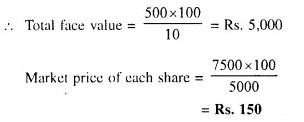Hope given Selina Concise Mathematics Class 10 ICSE Solutions Chapter 3 Shares and Dividend Ex 3A are helpful to complete your math homework.

If you have any doubts, please comment below. Learn Insta try to provide online math tutoring for you.

## Selina Concise Mathematics Class 10 ICSE Solutions Chapter 2 Banking (Recurring Deposit Accounts) Ex 2B

These Solutions are part of Selina Concise Mathematics Class 10 ICSE Solutions. Here we have given Selina Concise Mathematics Class 10 ICSE Solutions Chapter 2 Banking Ex 2B.

Other Exercises

Question 1.
Pramod deposits ₹ 600 per month in a Recurring Deposit Account for 4 years. If the rate of interest is 8% per year; calculate the maturity value of his account.
Solution:
Deposit per month (P) = ₹ 600
Rate of interest (r) = 8%
Period (n) = 4 years = 48 months.
According to formula,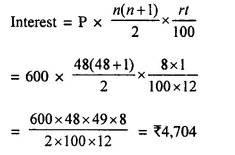Maturity value = ₹ 600 x 48 + ₹ 4,704 = ₹ 28,800 + ₹ 4,704 = ₹ 33504

Question 2.
Ritu has a Recurring Deposit Account in a bank and deposits ₹ 80 per month for 18 months. Find the rate of interest paid by the bank if the maturity value of this account is ₹ 1,554.
Solution:
Let rate of interest = r%,
n = 18,
P = ₹ 80
and A is maturity value.
Using formula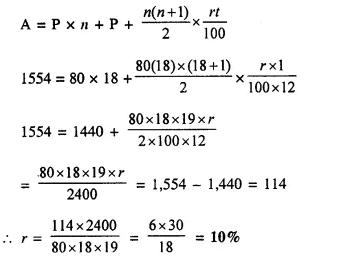Question 3.
The maturity value of a R.D. Account is ₹ 16,176. If the monthly installment is ₹ 400 and the rate of interest is 8%; find the time (period) of this R.D. Account.
Solution:
Here maturity value (A) = ₹ 16,176
Rate = 8%,
P = ₹ 400
Let period = n (No. of months)
Using formula :
I = A – P x n = 16,176 – 400 x n = 16,716 – 400n.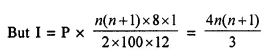⇒ 48,528 – 1,200n = 4n² + 4n
⇒ 4n² + 4n + 1200n – 48,528 = 0
⇒ 4n² + 1,204n – 48,528 = 0
⇒ n² + 301n — 12,132 = 0 (dividing by 4)
⇒ n² – 36n + 337n – 12,132 = 0
⇒ n (n – 36) + 337 (n – 36) = 0
⇒ (n – 36) (n + 337) = 0
Either n = 36 months or n = -337, which is not possible.
Time = 36 months = 3 years

Question 4.
Mr. Bajaj needs ₹ 30,000 after 2 years. What least money (in multiple of ₹ 5) must he deposit every month in a recurring deposit account to get required money at the end of 2 years, the rate of interest being 8% p.a. ?
Solution:
Amount of maturity = ₹ 30000
Period (n) = 2 years = 24 months
Rate = 8% p.a.
Let x be the monthly deposit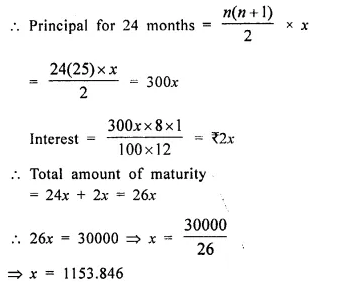Amount of monthly deposit in the multiple of ₹ 5 = ₹ 1155

Question 5.
Rishabh has a recurring deposit account in a post office for 3 years at 8% p.a. simple interest. If he gets ₹ 9,990 as interest at the time of maturity, find :
(i) the monthly installment.
(ii) the amount of maturity.
Solution:
Total interest = ₹ 9990
Period (n) = 3 years = 36 months
Rate of interest (r) = 8%
(i) Let monthly installment = x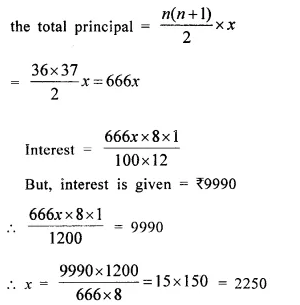Monthly installment = ₹ 2250
(ii) Amount of maturity = Principal + Interest
= 36 x 2250 + 9990
= ₹ 81000 + 9990 = ₹ 90990

Question 6.
Gopal has a cumulative deposit account and deposits ₹ 900 per month for a period of 4 years. If he gets ₹ 52,020 at the time of maturity, find the rate of interest.
Solution:
Maturity value = ₹ 52,020
Monthly installment (P) = ₹ 900
Total principal = ₹ 900 x 48 = ₹ 43200
Amount of interest = ₹ 52020 – ₹ 43200 = ₹ 8820
Let rate of interest = r%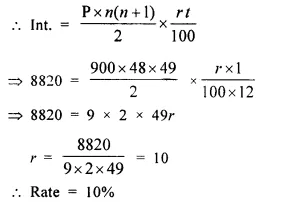Question 7.
Deepa has a 4 year recurring deposit account in a bank and deposits ₹ 1,800 per month. If she gets ₹ 1,800 per month. If she gets ₹ 1,08,450 at the time of maturity, find the rate of interest.
Solution:
Deposit per month = ₹ 1800
Period = 4 years = 48 months
Maturity value = ₹ 108450
Total principal = ₹ 1800 x 48 = ₹ 86400
Amount of interest = ₹ 108450 – 86400 = ₹ 22050
Let r be the rate of interest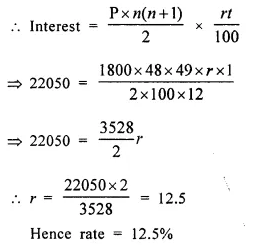Question 8.
Mr. Britto deposits a certain sum of money each month in a Recurring Deposit Account of a bank. If the rate of interest is of 8% per annum and Mr. Britto gets ₹ 8,088 from the bank after 3 years, find the value of his monthly installment. (2013)
Solution:
Let monthly installment = ₹ x
Period (n) = 3 x 12 months = 36 months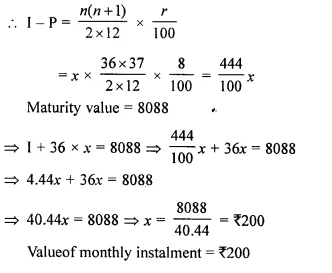Question 9.
Sharukh opened a Recurring Deposit Account in a bank and deposited ₹ 800 per month for 1$$\frac { 1 }{ 2 }$$ years. If he received ₹ 15,084 at the time of maturity, find the rate of interest per annum. (2014)
Solution:
Money deposited per month (P) = ₹ 800
r = ?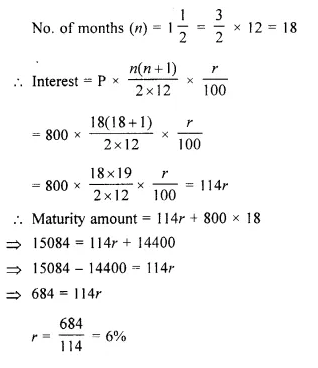Question 10.
Katrina opened a recurring deposit account with a Nationalised Bank for a period of 2 years. If the bank pays interest at the rate of 6% per annum and the monthly installment is ₹ 1,000, find the :
(i) interest earned in 2 years
(ii) maturity value. (2015)
Solution:
Period (n) = 2 years = 2 x 12 = 24 months
Rate of interest (r) = 6%
Monthly installment (P) = ₹ 1000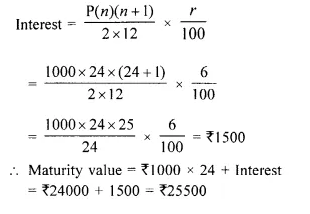Question 11.
Mohan has a recurring deposit account in a bank for 2 years at 6% p.a. simple interest. If he gets ₹ 1200 as interest at the time of maturity, find :
(i) the monthly installment
(ii) the amount of maturity
Solution:
(i) Interest = ₹ 1200,
n = 2 x 12 = 24 months,
r = 6%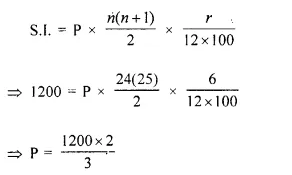⇒ P = ₹ 800
So the monthly installment is ₹ 800
(ii) Total sum deposited = P x n = ₹ 800 x 24 = ₹ 19200
The amount that Mohan will get at the time of maturity = Total sum deposited + Interest Received
= ₹ 19200 + ₹ 1200 = ₹ 20400
Hence, the amount of maturity is ₹ 20400

Hope given Selina Concise Mathematics Class 10 ICSE Solutions Chapter 2 Banking Ex 2B are helpful to complete your math homework.

If you have any doubts, please comment below. Learn Insta try to provide online math tutoring for you.